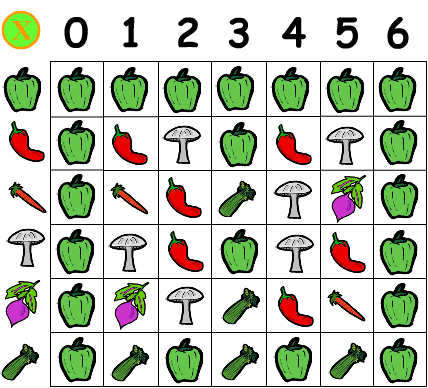### Background Notes for Teachers

This vegetables table is isomorphic to the modulo multiplication group Z(6), i.e. the six totatives of 7 multiplied modulo 7. carrot x carrot = chili pepper just as (3 x 3) modulo 7 = 9 mod 7 = 2.

N's totatives are strangers to N, meaning they're relatively prime or coprime to N, in addition to being less than N. For two numbers to be relatively prime, their greatest common divisor must be 1, i.e. a,b are coprime (strangers) if gcd(a,b)=1.

Group properties: Closure, Associativity, Inverses, Neutral element or identity (CAIN), with Abelian groups, named for Niels Henrik Abel, being commutative as well.

Whenever multiplication is modulo N over N's totatives, group properties obtain. It's not required that N be a prime. However, if N is prime, and we add 0, the additive identity, to the set (pick another vegetable), and define addition modulo N as well, then we get full-fledged finite field properties, i.e. Z(p) will be a commutative group for both addition and multiplication, plus the distributive law will hold.

Euler's totient function, often symbolized by phi, returns the number of N's totatives. If N is any prime p, then all positives less than p will have no factors in common with p (that's what it means to be prime) and so totient(p) = (p-1) for any prime. Note that we include 1 as a totative. Since 7 is prime, it has six totatives 1-6, which map to bell pepper, chili pepper, carrot, mushroom, beet and celery respectively.

The carrot would be equivalent to the number 3 and, as shown in the demo, has the distinction of being a generator of the group, meaning if you raise it to successive powers, you cycle through all the other vegetables. Do any of the other vegetables do that?

A useful exercise for students would be to create a table of powers, with the veggies down the left, and exponents 0 through 6 across the top. Define any vegetable to the 0th power to be the identity element, i.e. the bell pepper (the bell pepper times any element returns that element unchanged).Veggie Power!: Veggies to Powers 0-6

When this powers table is complete, you will notice that all vegetables to the sixth power equal the bell pepper (identity element). This result is expected according to Euler's Theorem, which asserts that raising any base b to the power of N's totient, modulo N, returns 1, provided gcd(b,N)=1.

Also note that all rows in the powers table define cyclic subgroups, whether or not they cycle through all members of the original group. Powers of chili peppers times powers of chili peppers give powers of chili peppers -- closure. You'll find inverses and a bell pepper (identity element) in every row of the powers table as well -- necessary conditions for grouphood.

Fermat's Little Theorem is a special case of this, when N is prime, i.e. base b to the (p-1) power mod p = 1, provided gcd(b,p)=1, i.e. b is not a multiple of p.

However, Fermat's Little Theorem (not to be confused with his more famous "last" theorem), does not assert that only primes obey this rule, merely that primes, among others, must obey it.

Indeed, some composite numbers do work in place of p, such that b to the (c-1) mod c = 1. Sometimes this works for some bases and not others (a real prime would work for any relatively prime base), and some work for all bases, these latter being the so-called Carmichael Numbers, named for their discoverer. 561 is the lowest Carmichael number.

For more on totatives and totients in multiplicative groups, see: Jiving in J, Crypto with Python.